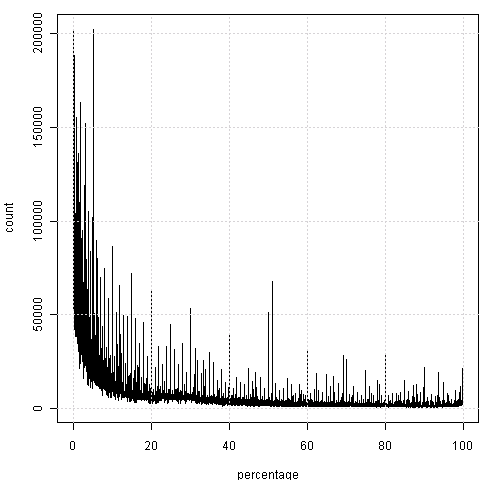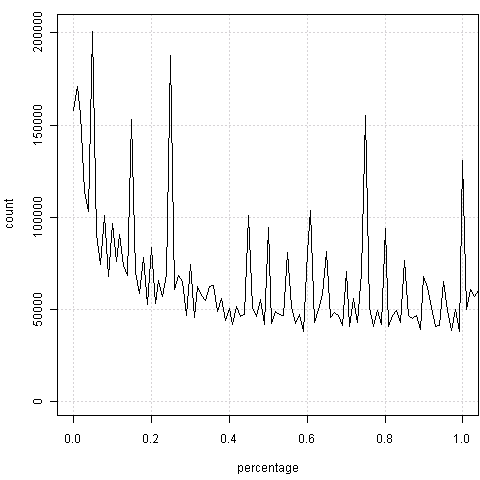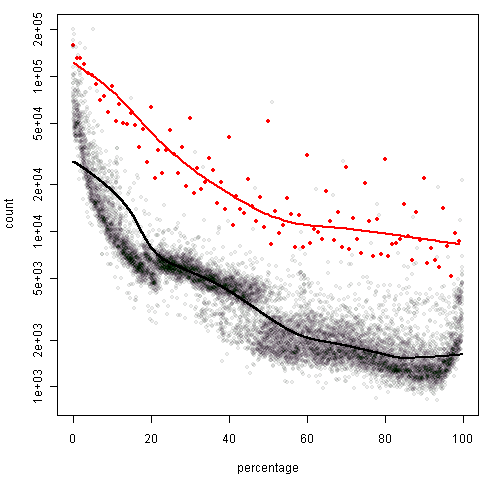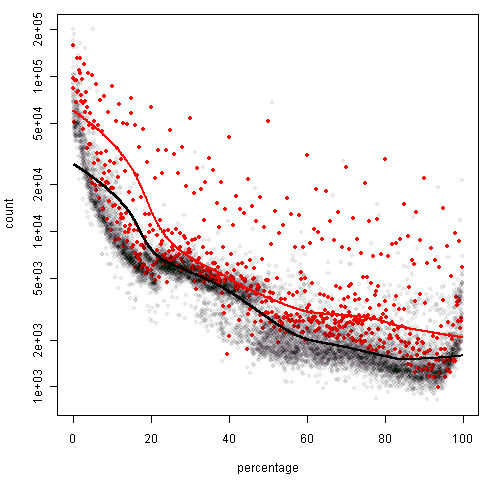# 杯具欣赏：他们眼中的统计学

### 谢益辉 2009-12-26

……在计算样本容量的时候要考虑一个置信区间的问题，也就是说调查了100个人，但是并不认为这100个人都是认真作答的，因此会在样本容量上再乘上一个置信度``````## 如果段炼不删掉这个数据的话，以下分析可重复
sep = ",", col.names = c("percentage", "count"))
x\$round0 = x\$percentage %in% seq(0, 100, 1)
x\$round1 = x\$percentage %in% seq(0, 100, 0.1)

plot(count ~ percentage, x, type = "l")
grid()

library(animation)
saveMovie({
for (i in 0:99) {
plot(count ~ percentage, x, type = "l", xlim = i + c(0,
1), panel.first = grid())
}
}, interval = 0.5, moviename = "percent-count",
para = list(mar = c(4.5, 4, 1, 0.1)))

plot(count ~ percentage, x, pch = 20, col = rgb(0:1,
0, 0, c(0.07, 1))[x\$round0 + 1], log = "y")
lines(lowess(x[x\$round0, 1:2], f = 1/3), col = "red", lwd = 2)
lines(lowess(x[!x\$round0, 1:2], f = 1/3), col = "black", lwd = 2)

plot(count ~ percentage, x, pch = 20, col = rgb(0:1,
0, 0, c(0.07, 1))[x\$round1 + 1], log = "y")
lines(lowess(x[x\$round1, 1:2], f = 1/3), col = "red", lwd = 2)
lines(lowess(x[!x\$round1, 1:2], f = 1/3), col = "black", lwd = 2)
``````